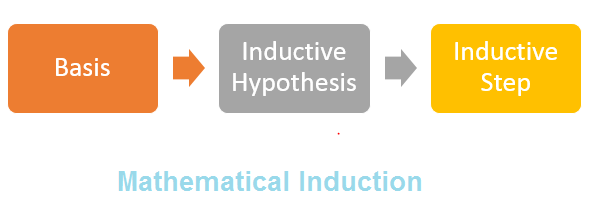# Principle of Mathematical Induction

Mathematical induction is a special technique used in Mathematics for proofs. It will be good to study deductive and inductive reasoning before learning it.

## What is Deductive reasoning

• Deductive reasoning also deductive logic or logical deduction or, informally, "top-down" logic is the process of reasoning from one or more statements (premises) to reach a logically certain conclusion
• Deductive reasoning" refers to the process of concluding that something must be true because it is a special case of a general principle that is known to be true. For example, if you know the general principle that the sum of the angles in any triangle is always 180 degrees, and you have a particular triangle in mind, you can then conclude that the sum of the angles in your triangle is 180 degrees.
• Deductive reasoning is logically valid and it is the fundamental method in which mathematical facts are shown to be true.

## What is Inductive reasoning

• It is the process of reasoning that a general principle is true because the special cases you have seen are true. For example, if all the people you have ever met from a particular town have been very strange, you might then say "all the residents of this town are strange". That is inductive reasoning: constructing a general principle from special cases.
• It goes in the opposite direction from deductive reasoning.
• Please don't confuse inductive reasoning with "mathematical induction" or and "inductive proof", which is something quite different

## The Principle of Mathematical Induction

Mathematical induction is a technique to prove statements for natural Numbers

How it works• Suppose there is a given statement P(n) involving the natural number n such that
• .
• Steps for Mathematical induction will be as below
(i) Shows The statement is true for n = 1, i.e., P(1) is true, and
(ii) Show that If the statement is true for n = k (where k is some positive integer), then
iii) the statement is also true for n = k + 1, i.e., truth of P(k) implies the truth of P (k + 1).
• .
• Important points
Step 1 is Basis of Induction
Step 2 is called inductive hypothesis. Here we are assuming the identity to be true as Step 1 is true(special case)
Step 3 is called inductive step. Here we prove the identity is true for k+1 on the basis of inductive hypothesis
So it is a deductive reasoning technique.

## Mathematical induction Solved Examples:

Question 1
Given n belongs to the set of Natural Numbers. Prove that using principle of Mathematical induction
$1 +2 +3 +4 + .....+ n=\frac {n(n+1)}{2}$
Solution:
Let us assume this statement as P(n)
Step 1 Basis of Induction
for P(0), we have LHS side as 0
RHS= 0(0+1)/2=0
So LHS=RHS

Step 2 inductive hypothesis
Let us assume P(n) is true,then
$1 +2 +3 +4 + .....+ n=\frac {n(n+1)}{2}$
Step 3 inductive step
Now we have to prove for P(n+1)
$1+2+......(n)+(n+1)=\frac{(n+1)[(n+1)+1]}{2}$

Now lets us take LHS
= [1+2+......(n)] +(n+1)
=$\frac{n(n+1)}{2}+(n+1)$ as P(n) is assumed to be true
=$\frac{(n+1)[(n+1)+1]}{2}$

So P(n+1) is true if P(n) is true. Now Since P(0) is true, we can say P(n) is good for all natural numbers

Question 2

To prove by mathematical induction that
6 (n+2) + 7(2n+1)
is divisible by 43 for each positive integer n.

Solution
Let P(n) be the statement
Step 1 Basis of Induction
P(1) =6 (1+1) + 7(2+1)
= 559 = 43 X 13,
the formula is true for n = 1.

Step 2 inductive hypothesis
Assume that P(n) is true for n = k, that is
P(k)=6 (k+2) + 7(2k+1)=43x
for some integer x.

Step 3 inductive step
Now show that the formula is true for n = k + 1.

Observe that
P(k+1)=6 (k+1+2) + 7(2k+2+1)

= 6(k+3) + 7(2k+3)
=6(k+2) + 727(2k+1)
=6X6(k+2) + (43+6)X7(2k+1)
=6X6(k+2) + 6X7(2k+1)+43X7(2k+1)
= 6 X ( P(k) ) + 43 X 7(2k+1)

Since each component of this sum is divisible by 43 so is the entire sum and the formula holds for k + 1

Question 3

Prove using Mathematical induction for $n \geq 1$
$1+4+7+......(3n-2)=\frac{n(3n-1)}{2}$
Solution
Let us assume this statement as P(n)
Step 1 Basis of Induction
Then P(1)
$1=\frac{1(3-1)}{2}$
Step 2 inductive hypothesis
Assume that P(n) is true for n = k, that is
$1+4+7+......(3k-2)=\frac{k(3k-1)}{2}$
Step 3 inductive step
Now show that the formula is true for n = k + 1.

Observe that

$1+4+7+.....[3(k+1)-2]=\frac{(k+1)[3(k+1)-1])}{2}$
Let us take the LHS
$1+4+7+.....[3(k+1)-2]=1+4+7+......+(3k-2)+(3k+1)$
$=\frac{k(3-1)}{2}+(3k+1)$
$=\frac{3k^{2}+5k+2}{2}$
$=\frac{(k+1)[3(k+1)+1]}{2}$
Question 4

Prove by the Principle of Mathematical Induction that
$1 \times 1! + 2 \times 2! + 3 \times 3! + ... + n \times n! = (n + 1)! - 1$ for all natural numbers n.
Solution
Let P(n) be the given statement
P(n) : $1 \times 1! + 2 \times 2! + 3 \times 3! + ... + n \times n! = (n + 1)!-� 1$ for all natural numbers n.
Note that P (1) is true, since
P (1) :$1 \times 1! = 1 = 2 - 1 = 2! - 1$.
Assume that P(n) is true for some natural number k, i.e.,
P(k) :$1 \times 1! + 2 \times 2! + 3 \times 3! + ... + k \times k! = (k + 1)! � 1$
To prove P (k + 1) is true, we have
P (k + 1) :$1 \times 1! + 2 \times 2! + 3 \times 3! + ... + k \times k! + (k + 1) \times (k + 1)!$
$= (k + 1)! - 1 + (k + 1)! \times (k + 1)$
$= (k + 1 + 1) (k + 1)! - 1$
$= (k + 2) (k + 1)! - 1 = ((k + 2)! - 1$
Hence Provded by Mathematical Induction